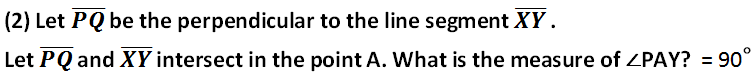# NCERT Class 6 Mathematics Fifth Chapter Understanding Elementary Shapes Exercise 5.5 Solutions

## EXERCISE 5.5

(1) Which of the following are models for perpendicular lines:

(a) The adjacent edges of a table top.

(c) The line segments forming the letter ‘L’.

In case you are missed :- Previous Chapter Exercise Solution(3) There are two set-squares in your box. What are the measures of the angles that are formed at their corners? Do they have any angle measure that is common?

(4) Study the diagram. The line l is perpendicular to line m

(a) Is CE = EG?

(b) Does PE bisect CG?

(c) Identify any two line segments for which PE is the perpendicular bisector.

(d) Are these true? (i) AC > FG (ii) CD = GH (iii) BC < EH.

In case you are missed :- Next Chapter Exercise Solution

Updated: April 14, 2023 — 2:05 pm